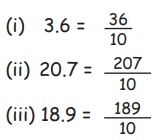Home | | Maths 5th Std | Relationship between Fractions and Decimals

# Relationship between Fractions and Decimals

Able to convert the fraction to decimals and vice versa

Relationship between Fractions and Decimals

To introduce the concept of decimal

Take a rectangle and spit the rectangle into 10 equal partsHow will you represent each part of the above rectangle?

That is 1/10, 1/10 and so on, Shade 1 part of the rectangle.Here 1 part is shaded out of 10 parts. Fractional representation is 1/10. It can be written in another way as 0.1 In 0.1 0 is called as the whole number part. 1 is called as the decimal part and “ “ is called as the decimal point which separates the unit place from tenth place.

The decimal number 0.1 is read as “ Zero tenth place”.

Able to convert the fraction to decimals and vice versa

To convert a fraction to a decimal, keep the point before the numerator as per the number of zeros in the denominator.Example 6.19

Convert the following decimals into fractions

i. 3.6

ii. 20.7

iii. 18.9

Solutioni. 3.6 = 36/10

ii. 20.7= 207/10

iii. 18.9 = 189/10

Tags : Fractions | Term 3 Chapter 6 | 5th Maths , 5th Maths : Term 3 Unit 6 : Fractions
Study Material, Lecturing Notes, Assignment, Reference, Wiki description explanation, brief detail
5th Maths : Term 3 Unit 6 : Fractions : Relationship between Fractions and Decimals | Fractions | Term 3 Chapter 6 | 5th Maths